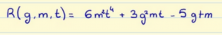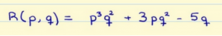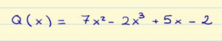Smartick is an advanced online program that teaches kids math and coding in only 15 min. a day

Apr26

# Review the Properties of Polynomials

In this post we will look at the properties of polynomials, what they are, what degree they have, and whether or not they complete and/or in standard form.

Let’s start by looking at what polynomials are.

A polynomial is an assembly of monomials.

Polynomials are usually named with capital letters (usually starting with the letter P) and the polynomial variables are written in parentheses.

P (a, b, c) = a 2 bc 3 – 3rd 3 c 6 + 6ac 4 + 2b

The polynomial P contains the variables a, b and c. It is composed of 4 monomials, therefore we can say that the polynomial has 4 terms.

Q (p, q) = -10p 6 + pq 2

The polynomial Q contains the variables p and q. It is composed of two monomials, therefore we can say that the polynomial has 2 terms.

### Degree of a polynomial

The degree of a polynomial is the largest of the degrees of their monomials.

Let’s look at the degrees of the above polynomials:

The polynomial P has 4 monomials:

a2bc3 –> Degree =6
-3a3c6  –> Degree = 9
6ac4  –> Degree = 5
2b –> Degree = 1

Therefore, the degree of the polynomial P is 9.

The polynomial Q has two monomials:

-10p6  –> Degree = 6
pq2  –> Degree = 3

Therefore, the polynomial Q has a degree of 6.

What do you think is the degree of this polynomial?### Polynomials in Standard Form

A polynomial is in standard form when its monomials are ordered from largest to smallest degree.

Returning to the previous polynomials, the polynomial P has monomials of degree 6, 9, 5, and 1. As they are not ordered from largest to smallest, we can say that the polynomial P is not in standard form.

The polynomial Q has the monomials of degrees: 6, 3. In this case the degrees of the monomials are ordered from largest to smallest degree, therefore the polynomial Q is in standard form.

Is the following polynomial ordered?### Complete Polynomials

A polynomial is complete when you have all terms with degrees from the largest to the zero degree.

The polynomial P containing degrees 6, 9, 5 and 1 is incomplete, because terms of degree 8, 7, 4, 3, 2 and 1 are missing.

The Q polynomial is also incomplete because it has terms of degree 6 and 3, thus missing terms of degree 5, 4, 2, 1 and 0.

A final exercise: Is this polynomial complete?With this we have finished the post for this week.

To keep learning, register now for a 15-day Smartick free trial!